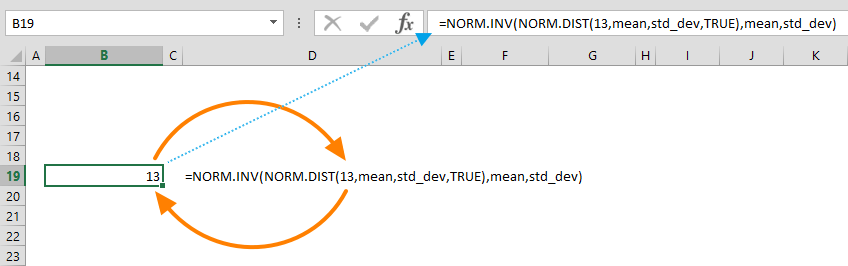The Excel NORM.INV (Also known as NORM INV or NORMINV) function is a Statistical formula that calculates and returns the inverse of the normal cumulative distribution of a probability for a given mean (average) and standard deviation. In this guide, we’re going to show you how to use the NORM.INV function and also go over some tips and error handling methods.

## Supported versions

• Excel 2010 and later (Earlier versions have support for the NORMINV function.)

## Excel NORM.INV Function Syntax

NORM.INV(x,mean,standard_dev)

## Arguments

 x The probability corresponding to the normal distribution. mean The arithmetic mean of the distribution. standard_dev The standard deviation of the distribution.

## NORM.INV Function Examples

The NORM.INV function calculates the value that satisfies the cumulative normal distribution function, based on the given mean and standard deviation values for that data set. Essentially, Excel uses the following approach, and returns the value for the probability as NORM.DIST(x, mean, standard_dev, TRUE) = probability.

=NORM.INV(0.11,26.44,11.17)=NORM.INV(NORM.DIST(13,mean,std_dev,TRUE),mean,std_dev) returns 13## Tips

• You can use the STDEV.P or STDEV.S functions to calculate the standard deviation for the entire population or a sample, respectively.
• You can use the AVERAGE function to calculate the mean of a data set.
• The NORMINV is the older version of this function. We recommend that you refrain from using the NORMINV function, unless you are using Excel 2010 or an older version that doesn't support NORM.INV.
• Other similar functions in the same family:
• NORM.DIST function returns the normal distribution.
• NORM.S.DIST function returns the standard normal distribution.
• NORM.S.INV function returns the inverse of the standard cumulative normal distribution.

## Issues

• The NORM.INV (NORM INV) function returns a #NUM! error value;
• If the standard_dev argument entered is ≤ 0
• If the probability ≤ 0 or if the probability ≥ 1